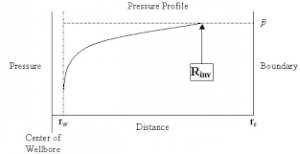## How to Calculate and Solve for the Radius of Investigation in Well Testing | The Calculator EncyclopediaThe image above represents radius of investigation.

To compute for the radius of investigation, five essential parameters are needed and these parameters are permeability (k), porosity (φ), viscosity (μ), time (t) and total compressibility (CT).

The formula for calculating the radius of investigation:

rinv = 0.0325 √[Kt / φμCT]

Where:

K = Permeability
φ = Porosity
μ = Viscosity
t = Time
CT = Total Compressibility

Let’s solve an example;
Find the radius of investigation when the permeability is 25, porosity is 18, viscosity is 12, time is 22 and total compressibility is 37.

This implies that;

K = Permeability = 25
φ = Porosity =18
μ = Viscosity = 12
t = Time = 22
CT = Total Compressibility = 37

rinv = 0.0325 √[Kt / φμCT]
rinv = 0.0325 √[25 x 22 / 18 x 12 x 37]
rinv = 0.0325 √[25 x 22 / 7992]
rinv = 0.0325 √[550 / 7992]
rinv = 0.0325 √[0.068]
rinv = 0.0325 [0.26]
rinv = 0.0085

Therefore, the radius of investigation is 0.0085 ft.